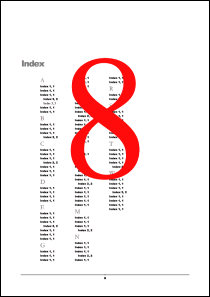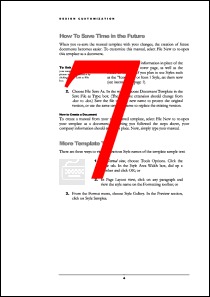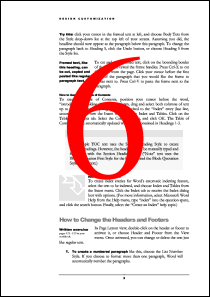RemapPages MethodRemaps pages for reordering, copying and deletion.Syntax

 [C#] ```void RemapPages(string pages) void RemapPages(int[] pages) void RemapPages(int[] pages, int index, int count)``` [Visual Basic] ```Sub RemapPages(pages As String) Sub RemapPages(pages() As Integer) Sub RemapPages(pages() As Integer, index As Integer, count As Integer)```Params

 Name Description pages The list of page numbers. index The index of the first page number into the array pages. count The number of page numbers in the array pages to use.Notes

 This method provides a simple method for remapping the pages in a document. It can be used for reordering, copying or deleting pages. It accepts a list of page numbers and reorders the pages in the document so that they match these page numbers. If a number is repeated more than once, the page is duplicated. If a number is omitted, the page is deleted. Page numbers can be delimited using spaces, commas or semicolons. The first page in a document is page one. So to reverse a four page document, you might use "4 3 2 1". If a page is duplicated and it contains Form fields, you may want to call MakeFieldsUnique to avoid sharing fields across pages.Example

The following code snippet illustrates how one might reverse all the pages in a document.

[C#]
```Doc theDoc = new Doc(); theDoc.Read(Server.MapPath("../mypics/sample.pdf")); theDoc.FontSize = 500; theDoc.Color.String = "255 0 0"; theDoc.TextStyle.HPos = 0.5; theDoc.TextStyle.VPos = 0.3; int theCount = theDoc.PageCount; string thePages = ""; for (int i = 1; i <= theCount; i++) {   theDoc.PageNumber = i;   theDoc.AddText(i.ToString());   thePages = thePages + (theCount - i + 1).ToString() + " "; } theDoc.RemapPages(thePages); theDoc.Save(Server.MapPath("docremappages.pdf")); theDoc.Clear();```
[Visual Basic]
```Dim theDoc As Doc = New Doc() theDoc.Read(Server.MapPath("../mypics/sample.pdf")) theDoc.FontSize = 500 theDoc.Color.String = "255 0 0" theDoc.TextStyle.HPos = 0.5 theDoc.TextStyle.VPos = 0.3 Dim theCount As Integer = theDoc.PageCount Dim thePages As String = "" For i As Integer = 1 To theCount   theDoc.PageNumber = i   theDoc.AddText(i.ToString())   thePages = thePages + (theCount - i + 1).ToString() + " " Next theDoc.RemapPages(thePages) theDoc.Save(Server.MapPath("docremappages.pdf")) theDoc.Clear()```docremappages.pdf [Page 1]docremappages.pdf [Page 2]docremappages.pdf [Page 3]docremappages.pdf [Page 4]